View: Next message | Previous More Hitsmessage Next in topic | Previous More Hitsin topic Next by same author | Previous More Hitsby same author Previous page (October 2001) | Back to main LRNASST-L page Join or leave LRNASST-L (or change settings) Reply | Post a new message Search Log in Options: Chronologically | Most recent first Proportional font | Non-proportional font

Subject:Re: Accounting for Perkins funds

From:Date:Tue, 16 Oct 2001 16:08:17 -0400

Content-Type:text/plain

Parts/Attachments: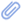text/plain (226 lines)
 ```Dear Catherine, Yes! We have also found that by maintaining two different timesheets, we can tap into the resources of the Perkins Vocational Grant. Makes great dollars and cents sense!:-) Take care, Sandie At 08:39 AM 10/16/01 -0700, you wrote: >This is a MIME message. If you are reading this text, you may want to >consider changing to a mail reader or gateway that understands how to >properly handle MIME multipart messages. > >--=_0F55F5C4.1A7B116E >Content-Type: text/plain; charset=US-ASCII >Content-Transfer-Encoding: quoted-printable > >We have our tutors use two different time sheets. As Tutor Coordinator, = >my job is to maintain a list of students using our tutoring services, = >including their major program of study. I make a list of those who are in = >a professional-technical major (Carl Perkins fund-approved), and when our = >tutors work with a student on that list, they record the time on their = >Perkins time sheet. I post the list where all tutors see it and update it = >weekly. It's extra work but worth it to have access to these funds. > >Catherine Quinnett, Tutor Coordinator >Linn-Benton Community College >Albany, OR >[log in to unmask] >=20 > >>>> [log in to unmask] 10/15/01 04:16PM >>> >Greetings, Listservers, >First, apologies for the length of this post. This question is for anyone >who receives Perkins funds -- the rest of you can hit "delete" and move on >:-). > >Our center has been getting Perkins \$\$ for a number of years, much of it = >for >tutoring. In the past, the focus for our funding was to serve vocational >students in special populations (students with Limited English Proficiency >[LEP] and students with disabilities). We simply used the funds to pay = >our >tutors, who were available to work with vocational students in these = >special >pops, as well as non-vocational and non-special pops students. Now, >however, Perkins is said to be changing. The signals we're getting = >indicate >less focus on special pops and a stronger emphasis on precise >accountability, which seems to be everyone's favorite term these days. In >other words, it looks like we need to be able to DOCUMENT that these funds >are being used ONLY to serve vocational students. Nobody seems exactly = >sure >what is expected, however. > >For us, this presents a logistical nightmare for a number of reasons. A >considerably larger proportion of our students are liberal arts rather = >than >vocational majors. We have a tutoring staff of about 35, and a given = >tutor >may well be tutoring mostly, a few, one, or no vocational students. Each = >of >the tutors keeps a timesheet which specifies the funding source. If we = >are >only allowed to use Perkins \$\$ to pay tutors when they are tutoring >vocational majors, this could mean having each tutor keep TWO timesheets, >recording tutoring sessions with non-voc students on one (using other >funds), and sessions with vocational students on the other (using Perkins >funds). Many tutors may not even KNOW the major of a student they are >tutoring in, say, English or Logic. So to expect them to keep track of >which tutee is and which tutee isn't a vocational major, and to record the >times for each on separate timesheets....!!! > >One proposed solution was "after-the-fact" accounting in which we'd track >tutoring sessions of vocational students and then transfer \$\$ from Perkins >to cover it later; unfortunately, it looks like this is not an option. >Alas, we are caught in a rather inflexible bureaucracy (polite >understatement). > >I hope I've explained this clearly enough. If it sounds convoluted, that's >probably because it is. How have others dealt with the need to show = >Perkins >\$\$ are being used in accordance with funding guidelines? > >Thanks in advance for any ideas! > >=3D=3D=3D=3D=3D=3D=3D=3D=3D=3D=3D=3D=3D=3D=3D=3D=3D=3D=3D=3D=3D=3D=3D=3D=3D= >=3D=3D=3D=3D=3D=3D=3D=3D=3D=3D=3D=3D=3D=3D=3D=3D=3D=3D=3D=3D=3D=3D=3D=3D=3D= >=3D=3D=3D >Beth Kupper-Herr >Associate Professor >Acting Coordinator, Learning Resource Center >Leeward Community College >96-045 Ala Ike >Pearl City, HI 96782 > >e-mail: [log in to unmask] >phone/voice mail: (808) 455-0413 >=3D=3D=3D=3D=3D=3D=3D=3D=3D=3D=3D=3D=3D=3D=3D=3D=3D=3D=3D=3D=3D=3D=3D=3D=3D= >=3D=3D=3D=3D=3D=3D=3D=3D=3D=3D=3D=3D=3D=3D=3D=3D=3D=3D=3D=3D=3D=3D=3D=3D=3D= >=3D=3D=3D > >--=_0F55F5C4.1A7B116E >Content-Type: text/html; charset=ISO-8859-1 >Content-Transfer-Encoding: quoted-printable >Content-Description: HTML > > > >> > >2px"> >
We have our tutors use two different time sheets. = > As=20 >Tutor Coordinator, my job is to maintain a list of students using our = >tutoring=20 >services, including their major program of study.  I make a list of = >those=20 >who are in a professional-technical major (Carl Perkins fund-approved), = >and when=20 >our tutors work with a student on that list, they record the time on = >their=20 >Perkins time sheet.  I post the list where all tutors see it = >and=20 >update it weekly.  It's extra work but worth it to have access to = >these=20 >funds.
>

>
Catherine Quinnett, Tutor Coordinator
>
Linn-Benton Community College
>
Albany, OR
>
>

Greetings, Listservers,
First, apologies for the length = >of=20 >this post.  This question is for anyone
who receives Perkins funds = >--=20 >the rest of you can hit "delete" and move on
:-).

Our center has = >been=20 >getting Perkins \$\$ for a number of years, much of it for
tutoring. = > In=20 >the past, the focus for our funding was to serve vocational
students = >in=20 >special populations (students with Limited English Proficiency
[LEP] = >and=20 >students with disabilities).  We simply used the funds to pay=20 >our
tutors, who were available to work with vocational students in = >these=20 >special
pops, as well as non-vocational and non-special pops students.&n= >bsp;=20 >Now,
however, Perkins is said to be changing.  The signals we're = >getting=20 >indicate
less focus on special pops and a stronger emphasis on=20 >precise
accountability, which seems to be everyone's favorite term = >these=20 >days.  In
other words, it looks like we need to be able to = >DOCUMENT that=20 >these funds
are being used ONLY to serve vocational students. Nobody = >seems=20 >exactly sure
what is expected, however.

For us, this presents = >a=20 >logistical nightmare for a number of reasons. A
considerably larger=20 >proportion of our students are liberal arts rather than
vocational=20 >majors.  We have a tutoring staff of about 35, and a given tutor
ma= >y=20 >well be tutoring mostly, a few, one, or no vocational students.  = >Each=20 >of
the tutors keeps a timesheet which specifies the funding source. = >; If=20 >we are
only allowed to use Perkins \$\$ to pay tutors when they are=20 >tutoring
vocational majors, this could mean having each tutor keep = >TWO=20 >timesheets,
recording tutoring sessions with non-voc students on one = >(using=20 >other
funds), and sessions with vocational students on the other = >(using=20 >Perkins
funds).  Many tutors may not even KNOW the major of a = >student=20 >they are
tutoring in, say, English or Logic.  So to expect them to = >keep=20 >track of
which tutee is and which tutee isn't a vocational major, and = >to=20 >record the
times for each on separate timesheets....!!!

One = >proposed=20 >solution was "after-the-fact" accounting in which we'd track
tutoring=20= > >sessions of vocational students and then transfer \$\$ from Perkins
to = >cover it=20 >later; unfortunately, it looks like this is not an option.
Alas, we = >are=20 >caught in a rather inflexible bureaucracy (polite
understatement).
R>I=20 >hope I've explained this clearly enough. If it sounds convoluted,=20 >that's
probably because it is.  How have others dealt with the = >need to=20 >show Perkins
\$\$ are being used in accordance with funding=20 >guidelines?

Thanks in advance for any=20 >ideas!

=3D=3D=3D=3D=3D=3D=3D=3D=3D=3D=3D=3D=3D=3D=3D=3D=3D=3D=3D=3D= >=3D=3D=3D=3D=3D=3D=3D=3D=3D=3D=3D=3D=3D=3D=3D=3D=3D=3D=3D=3D=3D=3D=3D=3D=3D= >=3D=3D=3D=3D=3D=3D=3D=3D
Beth=20 >Kupper-Herr
Associate Professor
Acting Coordinator, Learning = >Resource=20 >Center
Leeward Community College
96-045 Ala Ike
Pearl City, = >HI =20 >96782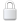Log In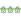Get Password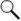Search Archives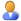Subscribe or Unsubscribe